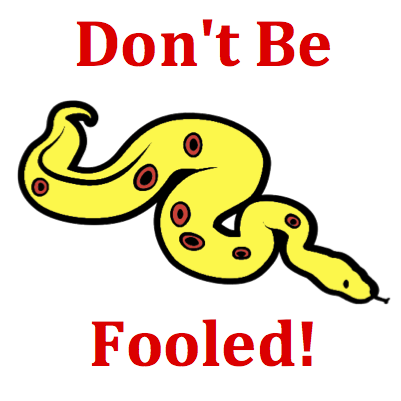# Kinematic Graphing - Mission KG6 Detailed HelpThe motion of an object is depicted by a velocity-time graph. Various time intervals during the motion are depicted by a letter. During which time interval(s) is the object moving with a negative velocity? List all that apply ... .Velocity-Time Graphs: Velocity versus time graphs represent changes that occur in an object's velocity with respect to time. The slope of the line is the acceleration (change in velocity divided by the change in time) of the object. The area under the line (between the line on the graph and the time axis) is the displacement of the object.Many students confuse position-time graphs with velocity-time graphs. They know that a negative velocity is represented on a position-time graph as a diagonal line with negative slope. So they instantly begin looking for the same line on this graph. But don't be fooled! This is a velocity-time graph and the slope is the acceleration. A line with negative slope on a velocity-time graph is a line with a negative acceleration.Many questions in this mission can be answered with a mixture of good math sense and logical thinking. The graph is a plot of velocity. There is a positive and a negative region displayed along the vertical axis. Any part of the graph that is being plotted below 0 m/s in the negative region has a negative velocity.